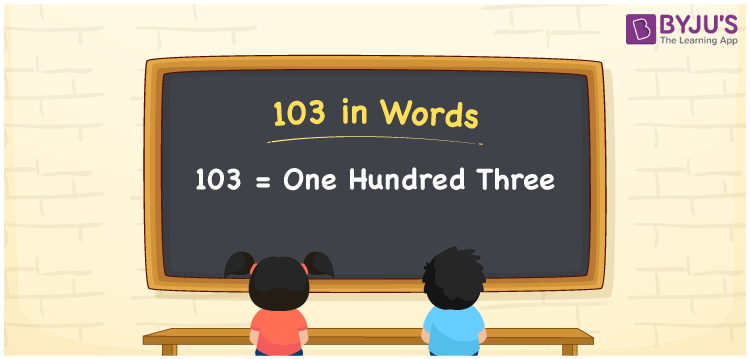# 103 in Words

103 in words is written as One Hundred Three. The name of number 103 in English is “One Hundred Three”. The word One Hundred Three is used in many real-life situations. For example, there are One Hundred Three students in a music class. Thus, it is a counting number. Also, to represent the currency equal to 103, we can write it in words as Rupees One Hundred Three or One Hundred Three rupees. Therefore, it is necessary to learn the numbers in words, for the ease of understanding and expressing them.

 103 in words One Hundred Three One Hundred Three in Numbers 103

## 103 in English Words## How to Write 103 in Words?

If we know the place value of digits of 103, then we can easily express it in words. The place value is basically the position of a digit in a number. 103 is a three-digit number, therefore, we can specify the position of each digit of 103 in a place value chart. In the Indian numbering system, the order of place value of digits from right to left is given by:

 Hundreds Tens Ones 1 0 3

We can write it in expanded form as:

1 × Hundred + 0 × Ten + 3 × One

= 1 × 100 + 0 × 10 + 3 × 1

= 100 + 3

= 103

= One Hundred Three

Therefore, 103 in words is written as eight hundred thirties.

103 is a natural number that precedes 104 and succeeds 102.

103 in words – One Hundred Three

Is 103 an odd number? – Yes

Is 103 an even number? – No

Is 103 a perfect square number? – No

Is 103 a perfect cube number? – No

Is 103 a prime number? – Yes

Is 103 a composite number? – No

## Frequently Asked Questions on 103 in Words

Q1

### How to write 103 in words?

103 in English is written as “One Hundred Three”.
Q2

### Is 103 an odd number or even number?

103 is an odd number because it is not wholly divisible by 2.
Q3

### Write One Hundred Three in numbers.

One Hundred Three in numbers is 103.